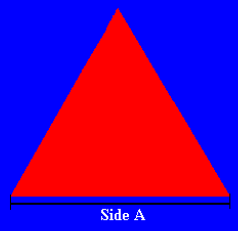# Equilateral Triangle Area and Perimeter CalculatorCalculate the area and perimeter of an equilateral triangle.

An equilateral triangle is a triangle with all three sides having an equal length.

Select unit:
Side A {{selectedunit.m1}}

#### Results:

 Area {{selectedunit.m1}}² Perimeter {{selectedunit.m1}}